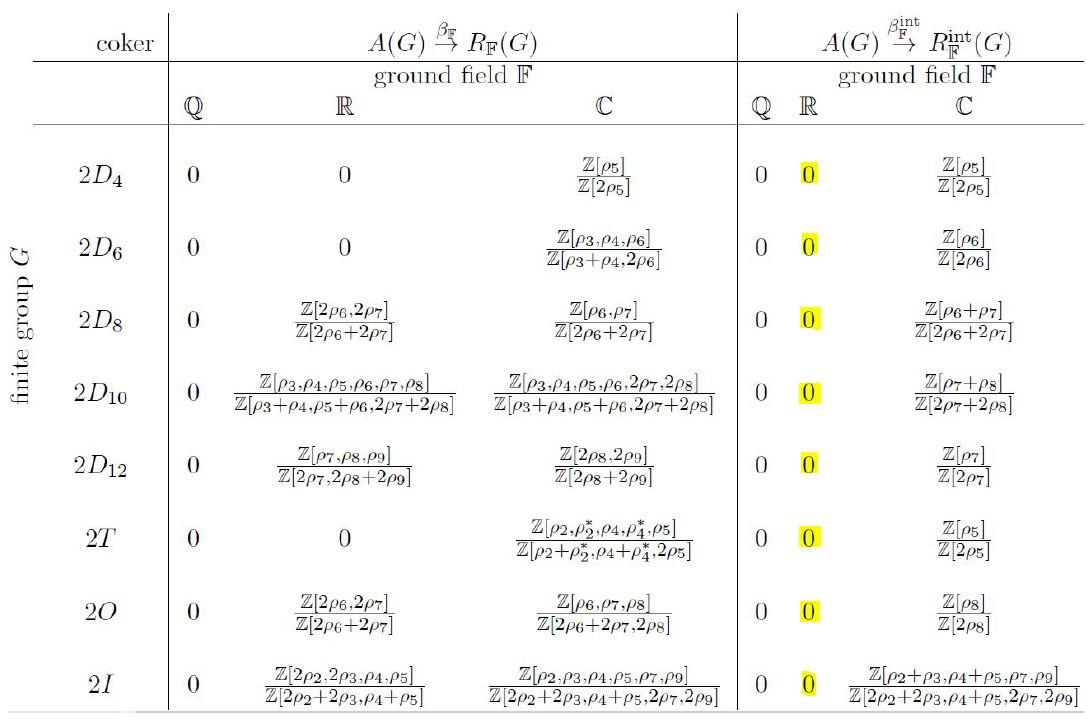Schreiber The image of the Burnside ring in the Representation ring

An article that we have written:• The image of the Burnside ring
in the Representation ring
– for binary Platonic groups

Abstract. We describe an efficient algorithm that computes, for any finite group $G$, the linear span of its virtual permutation representations inside all its linear representations, hence the image of the canonical morphism $A(G) \overset{\beta}{\longrightarrow} R_k(G)$ from the Burnside ring to the representation ring. We use this to determine the image and cokernel of $\beta$ for binary Platonic groups, hence for finite subgroups of SU(2), over $k \in \{\mathbb{Q}, \mathbb{R}, \mathbb{C}\}$. We find explicitly that for the three exceptional subgroups (2T, 2I, 2O) and for the first seven binary dihedral groups, $\beta$ surjects onto the sub-lattice $R^{\mathrm{int}}_{\mathbb{R}}(G)$ of the real representation ring spanned by the integer-valued characters. We conjecture that, generally, this holds true for all the binary dihedral groups.

Related articles

Related talks

Last revised on May 22, 2019 at 05:32:38. See the history of this page for a list of all contributions to it.